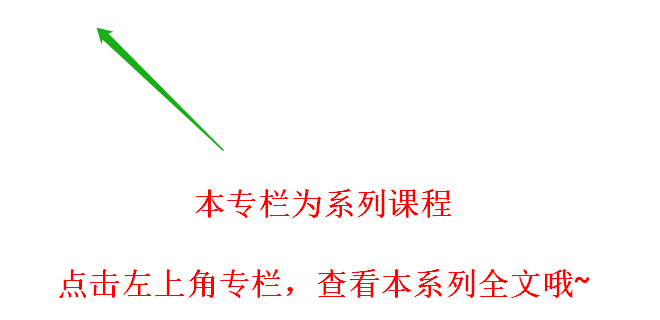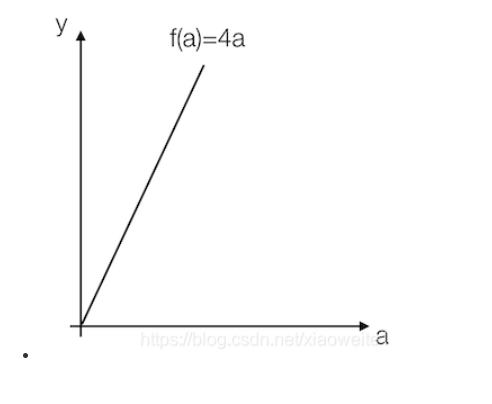# 搭建浅层神经网络"Hello world"

+关注继续查看

MNIST ("Modified National Institute of Standards and Technology")## 第一步：数据预处理

1.1查看数据标签

train = pd.read_csv('mnist/mnist_train.csv') # read train
test = pd.read_csv('mnist/mnist_test.csv') # read train

train.shape   （6000，785）
test.shape    （10000，785）1.2 提取数据标签

train_labels = np.array(train.pop('label'))
test_labels = np.array(test.pop('label'))## 第二步：数据可视化

2.1 随机生成数据匹配2.2 查看数据是否平衡

sns.distplot(train_labels, kde=False, bins=10)2.3 数据加载

# 图像宽度
width = 28
# 图像高度
height = 28
# batch size
batch_size = 100
# 训练图片数量
train_images_num = train.shape

train = tf.cast(train, tf.float32)
test = tf.cast(test, tf.float32)
train_ds = tf.data.Dataset.from_tensor_slices((train, train_labels)).shuffle(train_images_num).batch(batch_size)
test_ds = tf.data.Dataset.from_tensor_slices((test, test_labels)).batch(batch_size)

## 第三步：模型构建

3.1 构建模型的网络层次结构

• 第一层二维卷积层
• Flatten 层：这层的作用是将第一层的卷积曾平坦压缩成一维，常用在从卷积层到全连接曾的过度，当然 Flatten 不影响 batch 的大小
• Dense 层：全连接神经网络层
• Dense 层：全连接神经网络层

• 第一层使用 ReLU 函数
• Flatten 层（ 无 ）
• Dense 层 ReLU 函数
• Dense 层使用 softmax 损失函数进行输出

3.2 关于激活函数的解释说明

ReLU函数

ReLU 函数全名为线性整流函数（Rectified Linear Unit, ReLU），又称修正线性单元，是一种人工神经网络中常用的激活函数（activation function），通常指代以斜坡函数及其变种为代表的非线性函数。

ReLU的函数表达式为：• 收敛快
• 在[ 0, x ]区间内不会饱和，即它可以对抗梯度消失问题
• 求导简单，也就是它的计算效率很高

softmax 函数

softmax 用于多分类过程中，它将多个神经元的输出映射到（0,1）区间内，可以看成概率来理解，从而来进行多分类。class MNIST(Model):
def __init__(self):
super(MNIST, self).__init__()
self.conv1 = Conv2D(width, 3, activation='relu')
self.flatten = Flatten()
self.d1 = Dense(128, activation='relu')
self.d2 = Dense(10, activation='softmax')

def call(self, x):
x = self.conv1(x)
x = self.flatten(x)
x = self.d1(x)
return self.d2(x)

model = MNIST()
model.build(input_shape=train.shape

3.3 查看模型的构建情况batch 公式：loss_object = tf.keras.losses.SparseCategoricalCrossentropy()
optimizer = tf.keras.optimizers.SGD()
train_loss = tf.keras.metrics.Mean(name='train_loss')
train_accuracy = tf.keras.metrics.SparseCategoricalAccuracy(name='train_accuracy')

test_loss = tf.keras.metrics.Mean(name='test_loss')
test_accuracy = tf.keras.metrics.SparseCategoricalAccuracy(name='test_accuracy')

@tf.function
def train_step(images, labels):
predictions = model(images)
loss = loss_object(labels, predictions)

train_loss(loss)
train_accuracy(labels, predictions)

@tf.function
def test_step(images, labels):
predictions = model(images)
t_loss = loss_object(labels, predictions)
test_loss(t_loss)
test_accuracy(labels, predictions)

def lr_fn(epoch, lr):
if epoch == 0:
return 0.001

return lr * 0.9

## 第四步：模型训练plt.plot(epoch_range, train_accuracy_total, '-b', label= "training")
plt.plot(epoch_range, test_accuracy_total, '-g', label= "test")
plt.legend()
plt.xlabel('epoch')
plt.ylabel('accuracy')plt.plot(epoch_range, lr_total, '-b', label= "training")
plt.legend()
plt.xlabel('epoch')
plt.ylabel('learning rate')## 第五步：探索和优化

• 更深的网络层次，可以更换模型，比如使用 VGG16，ResNet 等更深的网络，或者在现有的网络中添加更多的卷积层进行尝试
• 更多的训练数据，数据量的增长能极大的提高模型的精度跟泛化能力
• 调整学习率

digit-recognizer 文件夹，矩池云已经将数据集和脚本都集成在其中，执行其中的 ipynb 文件，即可运行上述识别脚本。【Pytorch神经网络实战案例】19 神经网络实现估计互信息的功能

26 0【Pytorch神经网络实战案例】11 循环神经网络结构训练语言模型并进行简单预测

14 0【Pytorch神经网络实战案例】13 构建变分自编码神经网络模型生成Fashon-MNST模拟数据

30 0【Pytorch神经网络实战案例】12 利用注意力机制的神经网络实现对FashionMNIST数据集图片的分类

18 0【Pytorch神经网络实战案例】22 基于Cora数据集实现图注意力神经网络GAT的论文分类

28 0【Pytorch神经网络理论篇】 13 深层卷积神经网络介绍+池化操作+深层卷积神经网络实战
ceil_mode - 如果等于True，计算输出信号大小的时候，会使用向上取整，代替默认的向下取整的操作
26 0【Pytorch神经网络实战案例】33 使用BERT模型实现完形填空任务

25 0【Pytorch神经网络理论篇】 09 神经网络模块中的损失函数

14 099 045991